# MySQL数据库 || 增删改查操作详解

## 前言：

🎈增删改查是数据库的一些基本操作，我们要能够熟练掌握这些操作，并且能够理解其中的原理。这样我们对于数据库的操作就会得心应手。

## 插入数据

``````insert into person values(1,'唐僧',90,85.5,87),
-> (2,'八戒',50,60,87.6),
-> (3,'孙权',60,55,62.2),
-> (4,'曹操',50,56,70),
-> (5,'刘皇叔',70,75,62),
-> (6,'张飞',56,80,96);``````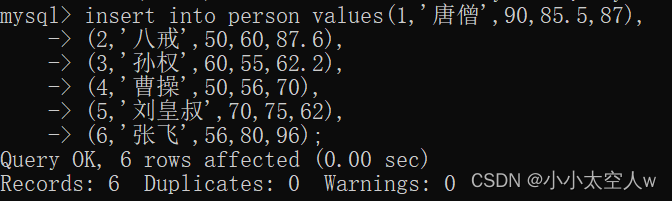注意：可以清楚看见数据已经插入成功。一次插入多条数据，比一次插入一条数据，代码执行效率要高。因为数据库是客户端服务器的结构，插入数据时，要通过网络，把数据写入服务器的硬盘中。可以想到一次性传输和一次性写入执行效率要比多次高。

## 查询数据

### 全列查询

``select * from person;``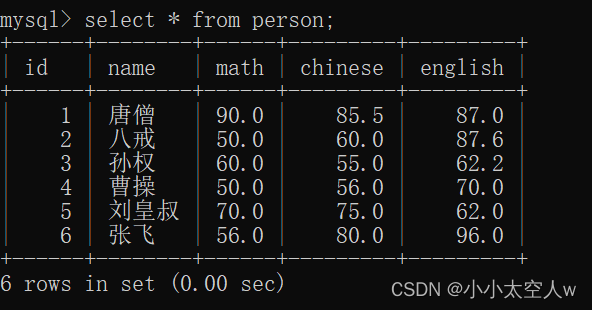### 指定列查询

`` select name,math from person;``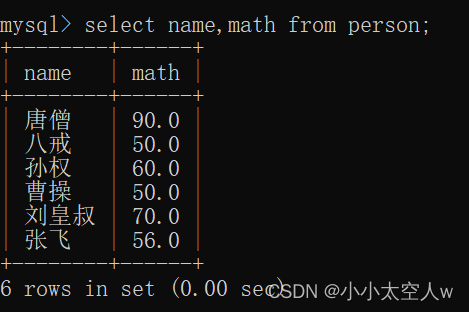### 带表达式查询

``select name,math + 10 from person;``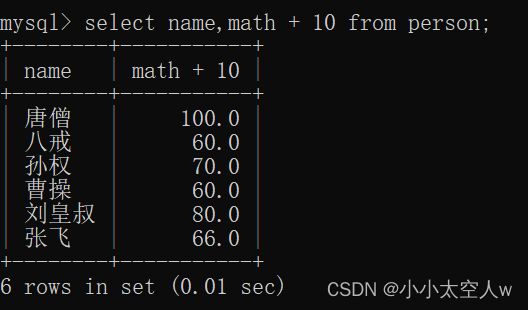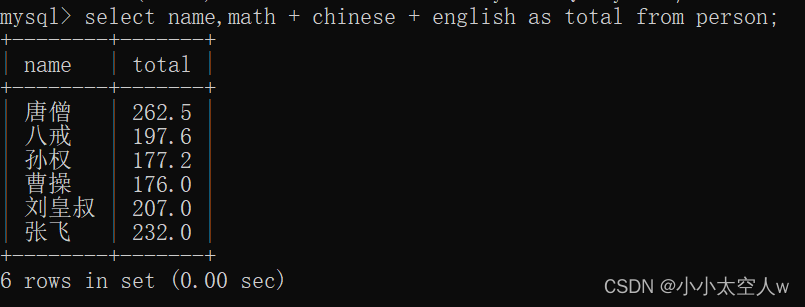### 去重查询

``````select distinct math from person;
select distinct math,chinese from person;``````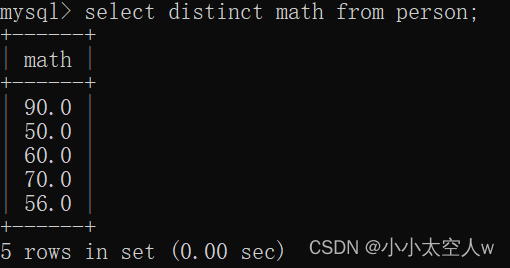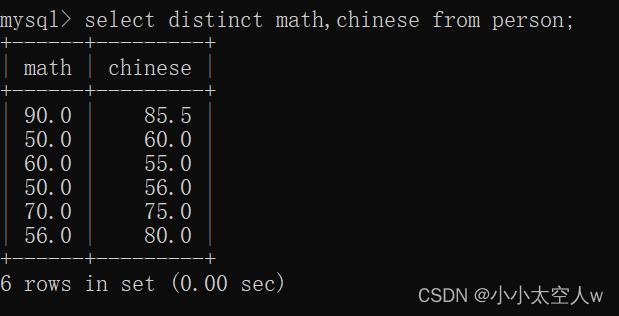注意：可以看见重复的50已经被去重。在指定多列时去重时，指定的每一列数据都一致才会被去重。

### 查询结果排序

``select name,math from person order by math;``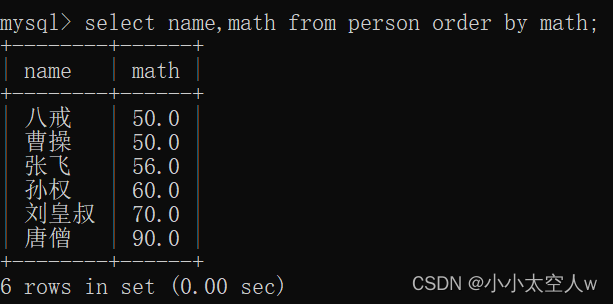``select name,math + chinese + english as total from person order by total;``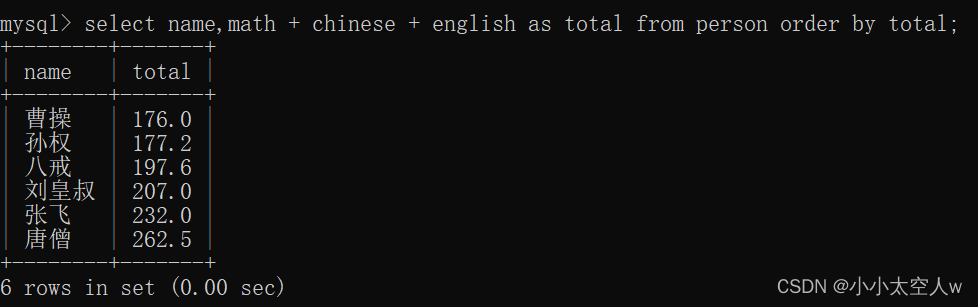多列排序

``````insert into person values(7,'孙悟空'，null,null,null);
select name,math,chinese from person order by math desc,chinese;``````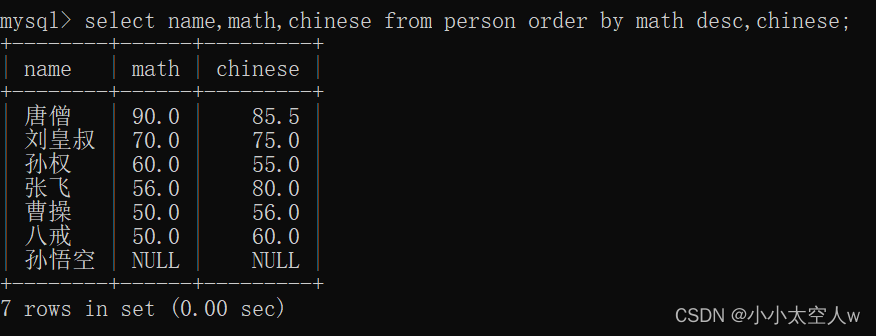### 条件查询

🤞条件查询会将每一条记录带入条件中，如果为true保留（作为临时表结构），为false舍弃。

🤞where后可带入表达式，但不能使用别名。MySQL中可能先执行条件判断部分，如果使用别名则会报错。

### 比较运算符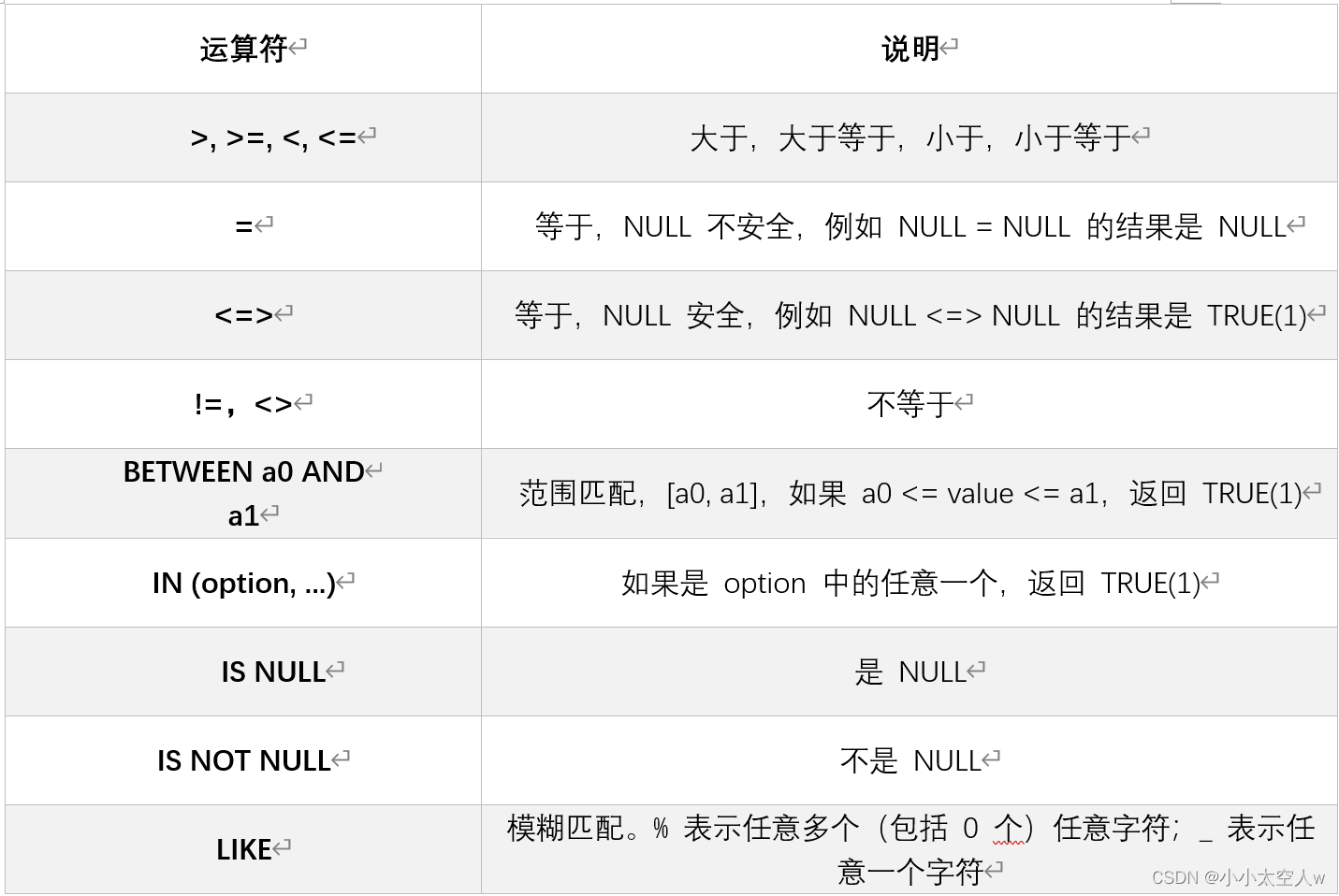### 逻辑运算符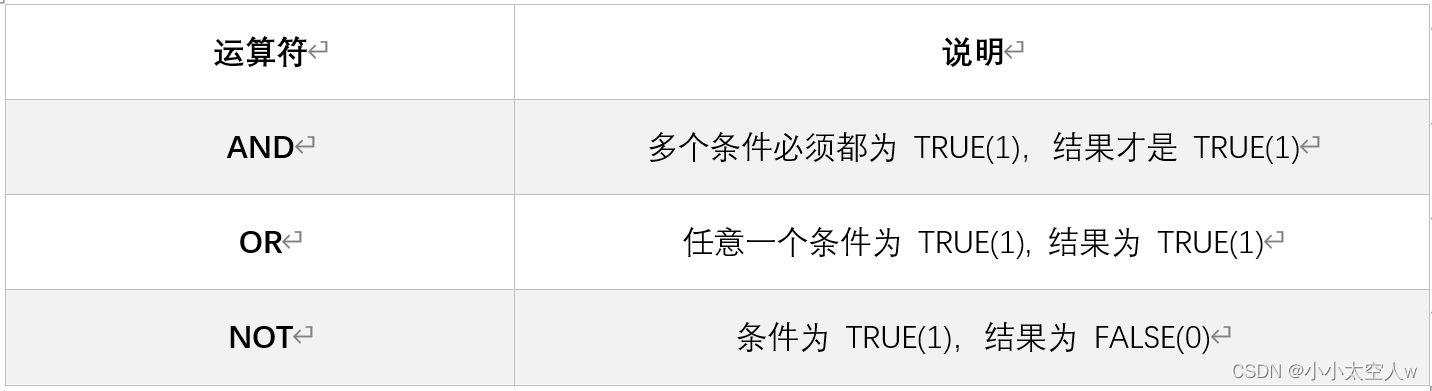🪖用null做运算结果都是null为false（除了特殊的<=>）。

🪖区间都是左闭右闭。

### 示例

数学成绩小于60

``select name,math from person where math < 60;``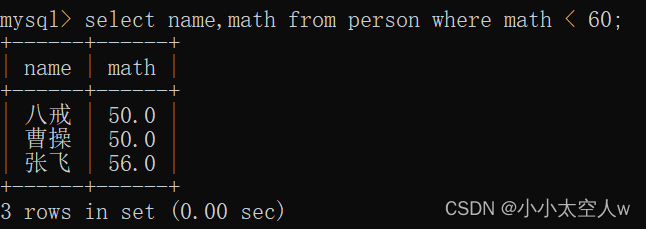数学成绩小于60包含null

`` select name,math from person where math < 60 or math is null;``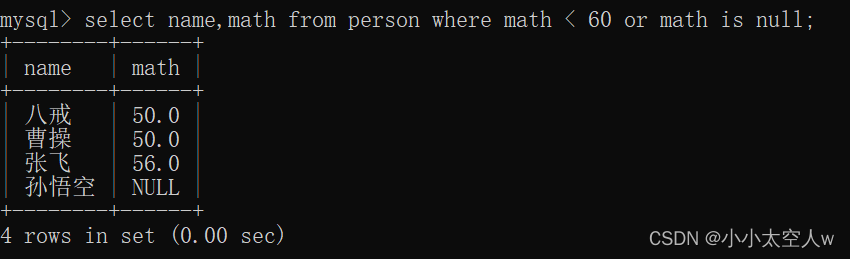`` select name,math,english from person where math > english;``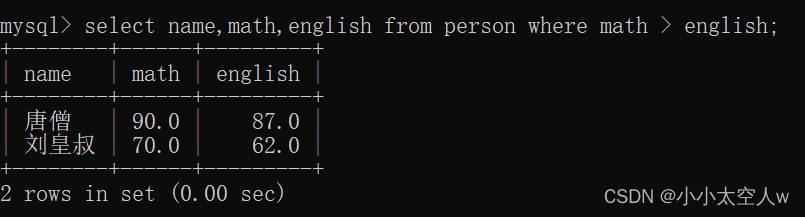总分在200分以上同学

``select name,math + chinese + english as total from person where math + chinese + english > 200``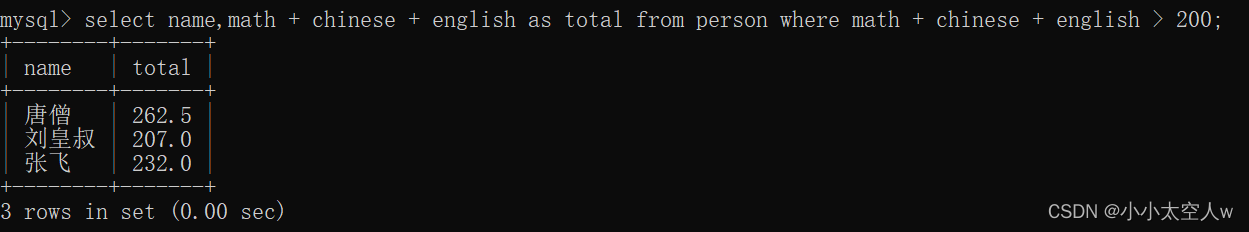数学大于80且语文大于80

``select name,math,chinese from person where math > 80 and chinese > 80;``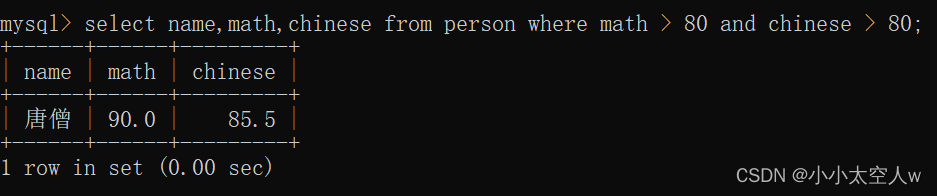数学大于80或者语文大于80（这里插入一条记录）

`````` insert into person values(8,'关羽',70,90,60);
select name,math,chinese from person where math > 80 or chinese > 80;``````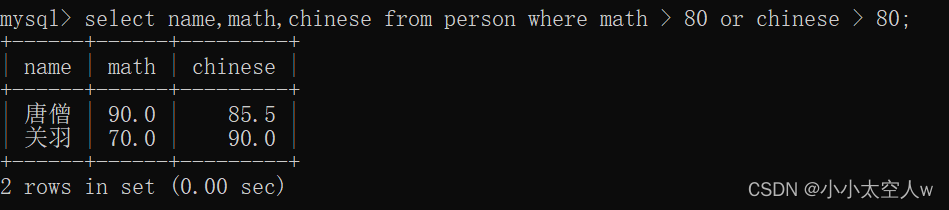``​​​​ select name,math from person where math between 60 and 80;``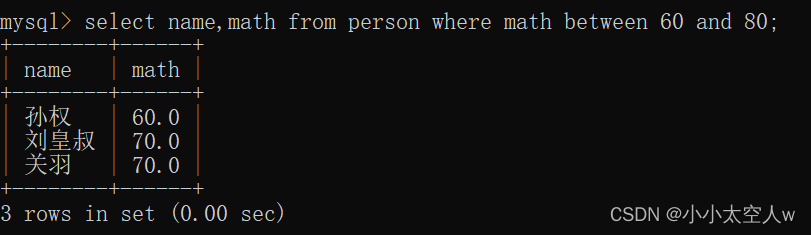数学成绩在60，70，80，90之中

`` select name,math from person where math in (60,70,80,90);``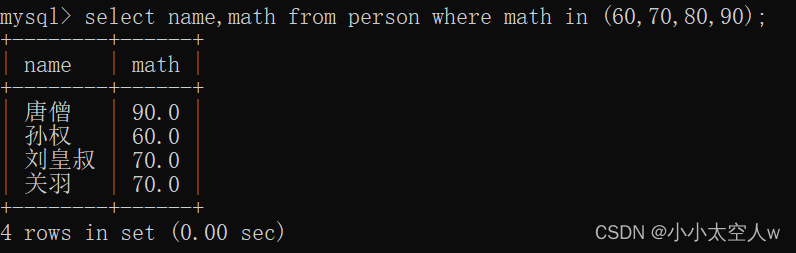### 示例

``````insert into person values(9,'哈哈孙',null,null,null);
insert into person values(10,'哈哈孙哈哈',null,null,null);
select * from person where name like '孙%';``````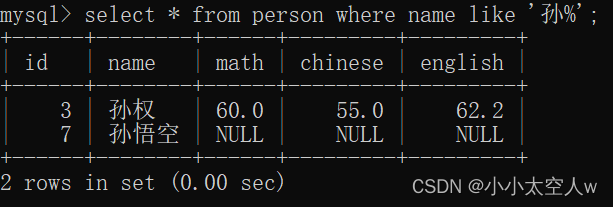最后一个字为孙

``select * from person where name like '%孙';``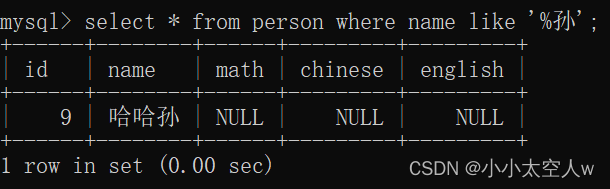``select * from person where name like '%孙%';``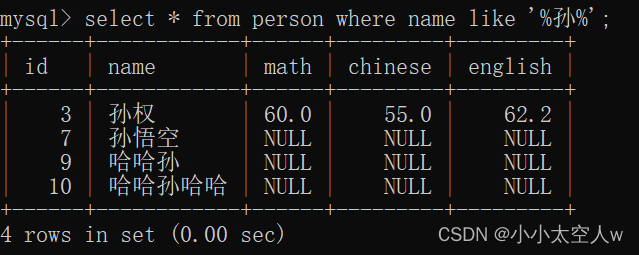``select * from person where name like '孙_';``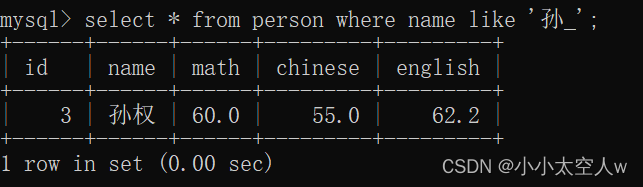``select * from person where name like '__孙';``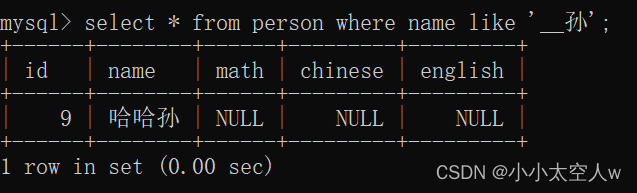### 空值比较

``select * from person where math is null;``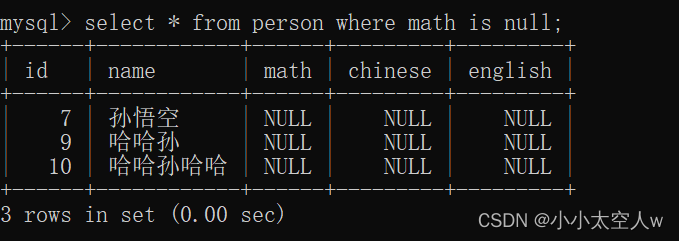``````insert into person values(11,'哈哈',80,80,70);
select * from person where math <=> chinese;``````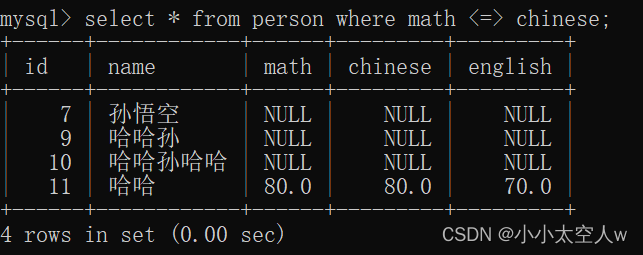### 分页查询

``select * from person limit 5;``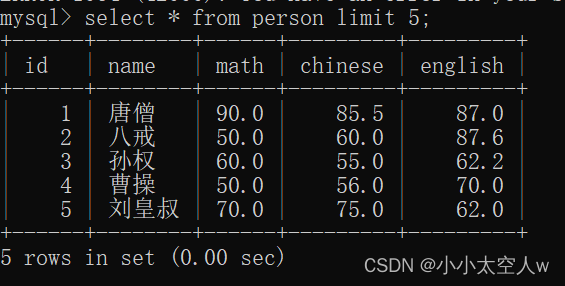``````select * from person limit 5 offset 0;
select * from person limit 5 offset 5;
select * from person limit 5 offset 10;``````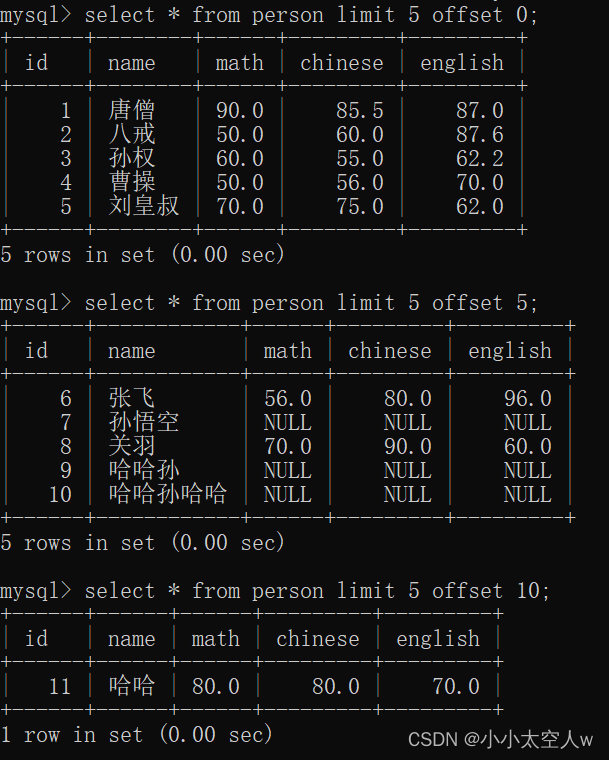## 修改数据

``update person set math = 0 where name = '孙悟空';``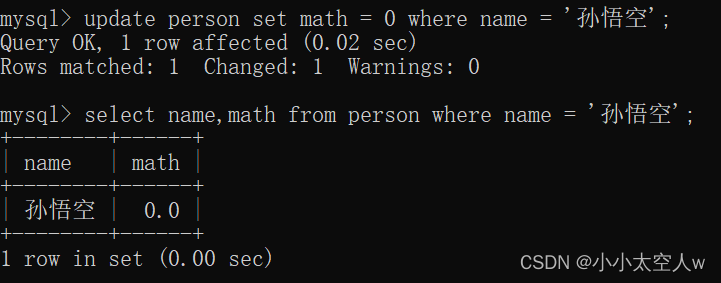`` update person set math = math + 5;``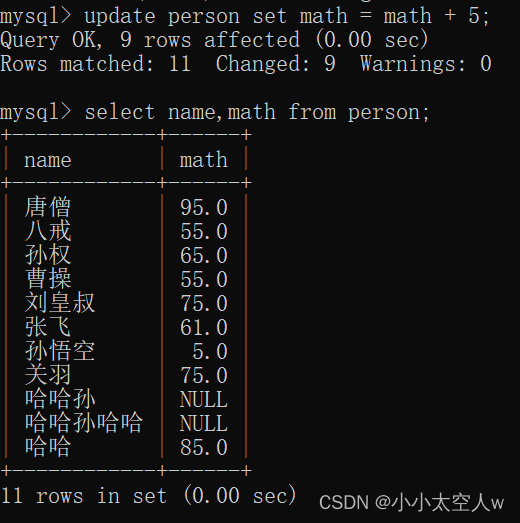``update person set math = 0,chinese = 0 where name = '孙悟空';``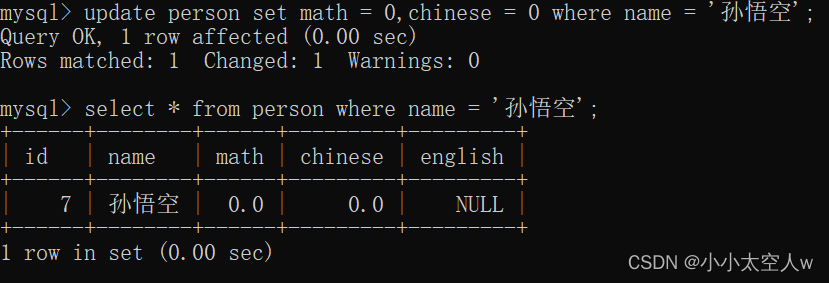``update person set math = 20 order by math + chinese + english limit 3;``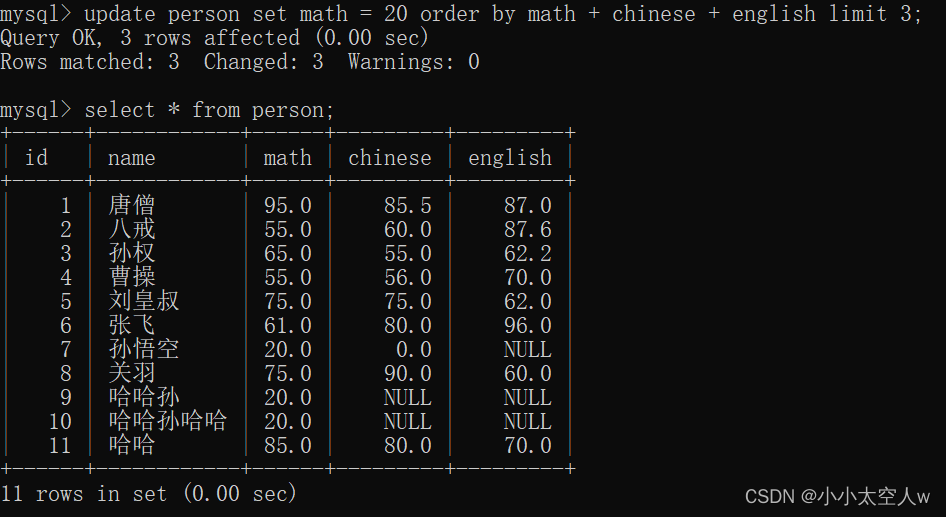## 删除数据

``delete from person where name = '孙悟空';``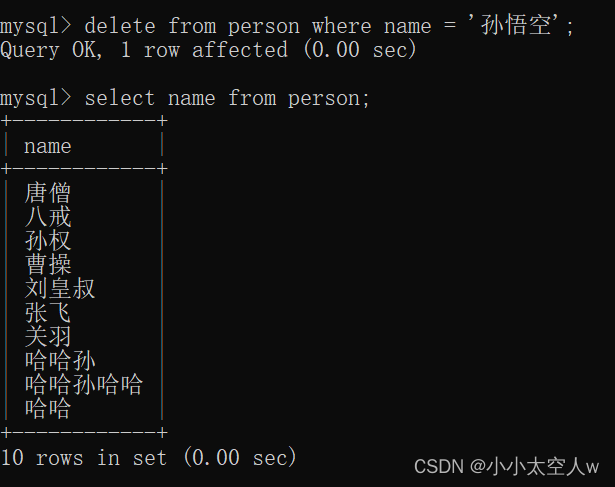删除表中所有记录（后面不写条件）

``delete from person;``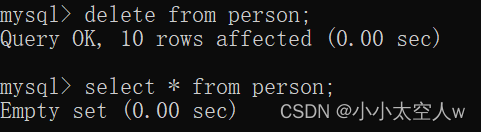## 注意：

🐵在学习数据库时需多练习，还需多去思考，这样会加深我们对其的理解性。